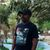App Development

Announcements
QlikWorld 2022, LIVE in Denver CO., May 16-19, 2022. REGISTER NOW TO RECEIVE EARLY BIRD PRICING
cancel
Showing results for
Did you mean:Contributor II

Qlik sense performance: slowlness issues IF statement

Hello,

I have a qlik sense application with many graphigs, in each graphics I used an expression with IF statement which causes a much slowness and some graphigs don't display sometimes.

if(GetSelectedCount(field1)=0 and GetSelectedCount(field2)=0 and GetSelectedCount(field3)=0,
Sum({<set analysis>} field4),
if(GetSelectedCount(field1)>0 and GetSelectedCount(field2)=0 and GetSelectedCount(field3)=0,
Sum({<setanalysis>} field4),
if(GetSelectedCount(field1)>=0 and (GetSelectedCount(field2)>0 and GetSelectedCount(field3)=0,
Sum({<set analysis>} field4),
)

I tried to implenement the conditions in the script but I don't know how replace the function: GetSelectedCount()

How can I avoid the use of IF or how can I replace it please?

Labels (2)

• slow performance

3 RepliesMaster II

This could probably be done with straight up set analysis, but I like mathematical solutions better than complicated set analysis, so I'd go about it that way personally. You should be able to do this with logical operators. Not sure if this will improve performance, since I haven't tried, but I think it should be efficient.

e.g.

(-(GetSelectedCount(Field1)=0 AND GetSelectedCount(Field2)=0 AND GetSelectedCount(Field3)=0)

* Sum({<set analysis>} field4))

+

(-(GetSelectedCount(Field1)>0 AND GetSelectedCount(Field2)=0 AND GetSelectedCount(Field3)=0)

* Sum({<other set analysis>} field4))

etc.

Each AND of this will return 0 for the conditional aspect if the conditions are not met, so that entire section will return 0 and not impact the result. It will return -1 (which we are turning into 1 using the negative sign) if all conditions are true, so we are multiplying by 1 and not changing the sum.Master

Try to check, the set analysis logic too and check the open , closed brackets b/w different conditions for where you were started it & ended it. If brackets () were not placed properly, selection won't pass properly, hence QlikSense not able to execute the proper set analysis condition.Contributor II
Author

Hi,

it is just an extract of my expression.

The expression works and the graphics display, I have just a problem of slowness.

I search to replace the if (string) by other way more performed.Tags
Community Browser